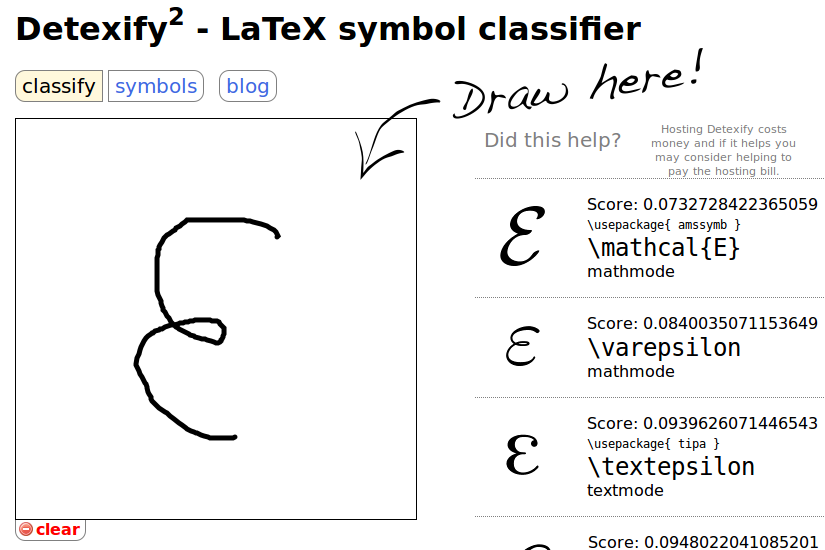# Mathjax与LaTex公式简介

## MathJax简介

MathJax是一款运行在浏览器中的开源的数学符号渲染引擎，使用MathJax可以方便的在浏览器中显示数学公式，不需要使用图片。目前，MathJax可以解析Latex、MathML和ASCIIMathML的标记语言。 MathJax项目于2009年开始，发起人有American Mathematical Society, Design Science等，还有众多的支持者，个人感觉MathJax会成为今后数学符号渲染引擎中的主流，也许现在已经是了。 本文接下来会讲述MathJax的基础用法，但不涉及MathJax的安装及配置。此外，推荐使用StackEdit学习MathJax的语法，它支持Markdown和MathJax，本文使用此编辑器撰写。

## 基础

### 希腊字母

alphaAAα\alpha
betaBBβ\beta
gammaΓ\Gammaγ\gamma
deltaΔ\Deltaδ\delta
epsilonEEϵ\epsilon
zetaZZζ\zeta
etaHHη\eta
thetaΘ\Thetaθ\theta
iotaIIι\iota
kappaKKκ\kappa
lambdaΛ\Lambdaλ\lambda
muMMμ\mu
nuNNν\nu
xiΞXiξ\xi
omicronOOο\omicron
piΠ\Piπ\pi
rhoPPρ\rho
sigmaΣ\Sigmaσ\sigma
tauTTτ\tau
upsilonΥ\Upsilonυ\upsilon
phiΦ\Phiϕ\phi
chiXXχ\chi
psiΨ\Psiψ\psi
omegaΩ\Omegaω\omega

### 括号

1. 小括号与方括号：使用原始的( )，[ ]即可，如(2+3)[4+4]：$(2+3)[4+4]$
2. 大括号：时由于大括号{}被用

1. 尖括号：使用\langle\rangle表示左尖括号和右尖括号。如\langle x \rangle：$\langle x \rangle$。
2. 上取整：使用\lceil\rceil表示。 如，\lceil x \rceil：$\lceil x \rceil$。
3. 下取整：使用\lfloor\rfloor表示。如，\lfloor x \rfloor：$\lfloor x \rfloor$。
4. 不可见括号：使用.表示。

\left \lbrace \sum_{i=0}^n i^2 = \frac{(n^2+n)(2n+1)}{6} \right\rbrace\tag{1.2}：$$\left \lbrace \sum_{i=0}^n i^2 = \frac{(n^2+n)(2n+1)}{6} \right\rbrace\tag{1.2}$$

### 求和与积分

\sum用来表示求和符号，其下标表示求和下限，上标表示上限。如，\sum_1^n：$\sum_1^n$。

\int用来表示积分符号，同样地，其上下标表示积分的上下限。如，\int_1^\infty：$\int_1^\infty$。

### 字体

1. 使用\mathbb\Bbb显示黑板粗体字，此字体经常用来表示代表实数、整数、有理数、复数的大写字母。如，$\mathbb{CHNQRZ}$。
2. 使用\mathbf显示黑体字，如，$\mathbf{ABCDEFGHIJKLMNOPQRSTUVWXYZ}$，$\mathbf{abcdefghijklmnopqrstuvwxyz}$。
3. 使用\mathtt显示打印机字体，如，$\mathtt{ABCDEFGHIJKLMNOPQRSTUVWXYZ}$，$\mathtt{abcdefghijklmnopqrstuvwxyz}$。
4. 使用\mathrm显示罗马字体，如，$\mathrm{ABCDEFGHIJKLMNOPQRSTUVWXYZ}$，$\mathrm{abcdefghijklmnopqrstuvwxyz}$。
5. 使用\mathscr显示手写体，如，$\mathscr{ABCDEFGHIJKLMNOPQRSTUVWXYZ}$。
6. 使用\mathfrak显示Fraktur字母（一种德国字体），如$\mathfrak{ABCDEFGHIJKLMNOPQRSTUVWXYZ}$，$\mathrm{abcdefghijklmnopqrstuvwxyz}$。

### 特殊函数与符号

1. 常见的三角函数，求极限符号可直接使用+缩写即可。如\sin x:$\sin x$,\arctan x:$\arctan x$,\lim_{1\to\infty}:$\lim_{1\to\infty}$。
2. 比较运算符：\lt \gt \le \ge \neq ： $\lt \gt \le \ge \neq$。可以在这些运算符前面加上\not，如\not\lt：$\not\lt$。
3. \times \div \pm \mp表示：$\times \div \pm \mp$，\cdot表示居中的点，x \cdot y : $x \cdot y$。
4. 集合关系与运算：\cup \cap \setminus \subset \subseteq \subsetneq \supset \in \notin \emptyset \varnothing ：$\cup \cap \setminus \subset \subseteq \subsetneq \supset \in \notin \emptyset \varnothing$.
5. 表示排列使用{n+1 \choose 2k}\binom{n+1}{2k}，${n+1 \choose 2k}$。
6. 箭头：\to \rightarrow \leftarrow \Rightarrow \Leftarrow \mapsto : $\to \rightarrow \leftarrow \Rightarrow \Leftarrow \mapsto$。
7. 逻辑运算符：\land \lor \lnot \forall \exists \top \bot \vdash \vDash ： $\land \lor \lnot \forall \exists \top \bot \vdash \vDash$。
8. \star \ast \oplus \circ \bullet ： $\star \ast \oplus \circ \bullet$。
9. \approx \sim \cong \equiv \prec ： $\approx \sim \cong \equiv \prec$。
10. \infty \aleph_0 $\infty \aleph_0$ \nabla \partial $\nabla \partial$ \Im \Re $Im \Re$。
11. 模运算 \pmode, 如，a\equiv b\pmod n：$a\equiv b\pmod n$。
12. \ldots\cdots，其区别是dots的位置不同，ldots位置稍低，cdots位置居中。$a_1+a_2+\cdots+a_n$，$a_1, a_2, \ldots ,a_n$。
13. 一些希腊字母具有变体形式，如 \epsilon \varepsilon : $\epsilon \varepsilon$, \phi \varphi $\phi \varphi$。

## 表格

$$\begin{array}{c|lcr} n & \text{Left} & \text{Center} & \text{Right} \\ \hline 1 & 0.24 & 1 & 125 \\ 2 & -1 & 189 & -8 \\ 3 & -20 & 2000 & 1+10i \\ \end{array}$$

$$\begin{array}{c|lcr} n & \text{Left} & \text{Center} & \text{Right} \\ \hline 1 & 0.24 & 1 & 125 \\ 2 & -1 & 189 & -8 \\ 3 & -20 & 2000 & 1+10i \\ \end{array}$$

$$% outer vertical array of arrays \begin{array}{c} % inner horizontal array of arrays \begin{array}{cc} % inner array of minimum values \begin{array}{c|cccc} \text{min} & 0 & 1 & 2 & 3\\ \hline 0 & 0 & 0 & 0 & 0\\ 1 & 0 & 1 & 1 & 1\\ 2 & 0 & 1 & 2 & 2\\ 3 & 0 & 1 & 2 & 3 \end{array} & % inner array of maximum values \begin{array}{c|cccc} \text{max}& 0& 1& 2& 3\\ \hline 0 & 0 & 1 & 2 & 3\\ 1 & 1 & 1 & 2 & 3\\ 2 & 2 & 2 & 2 & 3\\ 3 & 3 & 3 & 3 & 3 \end{array} \end{array} \\ % inner array of delta values \begin{array}{c|cccc} \Delta& 0& 1& 2& 3\\ \hline 0 & 0 & 1 & 2 & 3\\ 1 & 1 & 0 & 1 & 2\\ 2 & 2 & 1 & 0 & 1\\ 3 & 3 & 2 & 1 & 0 \end{array} \end{array}$$

## 矩阵

### 基本用法

$$\begin{matrix} 1 & x & x^2 \\ 1 & y & y^2 \\ 1 & z & z^2 \\ \end{matrix}$$

$$\begin{matrix} 1 & x & x^2 \\ 1 & y & y^2 \\ 1 & z & z^2 \\ \end{matrix}$$

### 省略元素

$$\begin{pmatrix} 1 & a_1 & a_1^2 & \cdots & a_1^n \\ 1 & a_2 & a_2^2 & \cdots & a_2^n \\ \vdots & \vdots& \vdots & \ddots & \vdots \\ 1 & a_m & a_m^2 & \cdots & a_m^n \end{pmatrix}$$

### 增广矩阵

$$\left[ \begin{array}{cc|c} 1&2&3\\ 4&5&6 \end{array} \right]$$

$$\left[ \begin{array}{cc|c} 1&2&3\\ 4&5&6 \end{array} \right]$$

## 对齐的公式

\begin{align} \sqrt{37} & = \sqrt{\frac{73^2-1}{12^2}} \\ & = \sqrt{\frac{73^2}{12^2}\cdot\frac{73^2-1}{73^2}} \\ & = \sqrt{\frac{73^2}{12^2}}\sqrt{\frac{73^2-1}{73^2}} \\ & = \frac{73}{12}\sqrt{1 - \frac{1}{73^2}} \\ & \approx \frac{73}{12}\left(1 - \frac{1}{2\cdot73^2}\right) \end{align}

## 分类表达式

$$f(n) = \begin{cases} n/2, & \text{if n is even} \\ 3n+1, & \text{if n is odd} \\ \end{cases}$$

$$\left. \begin{array}{l} \text{if n is even:}&n;/2\\ \text{if n is odd:}&3n+1 \end{array} \right\} =f(n)$$

## 数学符号查询## 空间问题

### 不要在再指数或者积分中使用 \frac

$$\begin{array}{cc} \mathrm{Bad} & \mathrm{Better} \\ \hline \\ e^{i\frac{\pi}2} \quad e^{\frac{i\pi}2}& e^{i\pi/2} \\ \int_{-\frac\pi2}^\frac\pi2 \sin x\,dx & \int_{-\pi/2}^{\pi/2}\sin x\,dx \\ \end{array}$$

### 使用 \mid 代替 | 作为分隔符

$$\begin{array}{cc} \mathrm{Bad} & \mathrm{Better} \\ \hline \\ \{x|x^2\in\Bbb Z\} & \{x\mid x^2\in\Bbb Z\} \\ \end{array}$$

### 多重积分

$$\begin{array}{cc} \mathrm{Bad} & \mathrm{Better} \\ \hline \\ \int\int_S f(x)\,dy\,dx & \iint_S f(x)\,dy\,dx \\ \int\int\int_V f(x)\,dz\,dy\,dx & \iiint_V f(x)\,dz\,dy\,dx \end{array}$$

$$\begin{array}{cc} \mathrm{Bad} & \mathrm{Better} \\ \hline \\ \iiint_V f(x)dz dy dx & \iiint_V f(x)\,dz\,dy\,dx \end{array}$$

## 连分数

$$x = a_0 + \cfrac{1^2}{a_1 + \cfrac{2^2}{a_2 + \cfrac{3^2}{a_3 + \cfrac{4^4}{a_4 + \cdots}}}} \tag{\cfrac}$$

$$x = a_0 + \frac{1^2}{a_1 + \frac{2^2}{a_2 + \frac{3^2}{a_3 + \frac{4^4}{a_4 + \cdots}}}} \tag{\frac}$$

## 方程组

$$\left\{ \begin{array}{c} a_1x+b_1y+c_1z=d_1 \\ a_2x+b_2y+c_2z=d_2 \\ a_3x+b_3y+c_3z=d_3 \end{array} \right.$$

$$\begin{cases} a_1x+b_1y+c_1z=d_1 \\ a_2x+b_2y+c_2z=d_2 \\ a_3x+b_3y+c_3z=d_3 \end{cases}$$

\left\{ \begin{aligned} a_1x+b_1y+c_1z & =d_1+e_1 \\ a_2x+b_2y& =d_2 \\ a_3x+b_3y+c_3z & =d_3 \end{aligned} \right.

$$\left\{ \begin{array}{ll} a_1x+b_1y+c_1z & =d_1+e_1 \\ a_2x+b_2y & =d_2 \\ a_3x+b_3y+c_3z & =d_3 \end{array} \right.$$

## 颜色

$$\begin{array}{|rc|} \hline \verb+\color{black}{text}+ & \color{black}{text} \\ \verb+\color{gray}{text}+ & \color{gray}{text} \\ \verb+\color{silver}{text}+ & \color{silver}{text} \\ \verb+\color{white}{text}+ & \color{white}{text} \\ \hline \verb+\color{maroon}{text}+ & \color{maroon}{text} \\ \verb+\color{red}{text}+ & \color{red}{text} \\ \verb+\color{yellow}{text}+ & \color{yellow}{text} \\ \verb+\color{lime}{text}+ & \color{lime}{text} \\ \verb+\color{olive}{text}+ & \color{olive}{text} \\ \verb+\color{green}{text}+ & \color{green}{text} \\ \verb+\color{teal}{text}+ & \color{teal}{text} \\ \verb+\color{aqua}{text}+ & \color{aqua}{text} \\ \verb+\color{blue}{text}+ & \color{blue}{text} \\ \verb+\color{navy}{text}+ & \color{navy}{text} \\ \verb+\color{purple}{text}+ & \color{purple}{text} \\ \verb+\color{fuchsia}{text}+ & \color{magenta}{text} \\ \hline \end{array}$$

$$\begin{array}{|rrrrrrrr|}\hline \verb+#000+ & \color{#000}{text} & & & \verb+#00F+ & \color{#00F}{text} & & \\ & & \verb+#0F0+ & \color{#0F0}{text} & & & \verb+#0FF+ & \color{#0FF}{text}\\ \verb+#F00+ & \color{#F00}{text} & & & \verb+#F0F+ & \color{#F0F}{text} & & \\ & & \verb+#FF0+ & \color{#FF0}{text} & & & \verb+#FFF+ & \color{#FFF}{text}\\ \hline \end{array}$$

HTML色彩快速参考手册

## 公式标记与引用

$$a := x^2-y^3 \tag{*}\label{*}$$

$$a+y^3 \stackrel{\eqref{*}}= x^2$$

Written with StackEdit.